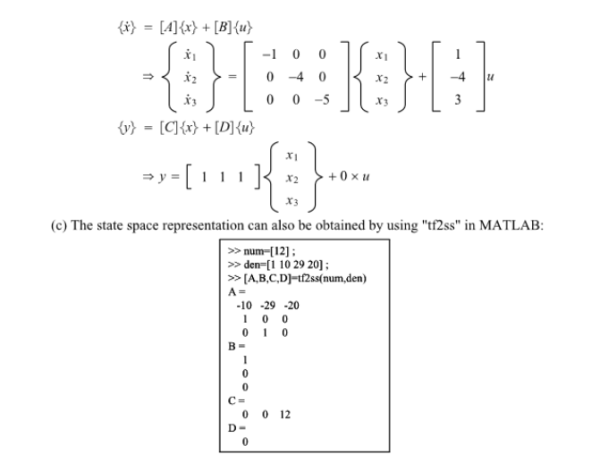For the given transfer function G(s)=Y/U, (a) Obtain a state space representation (including both state equation and output equation) using the following state variables: x1=y, x2=ydot, x3=ydoubledot. (b) Obtain a state space representation using the partial fraction expansion approach. (c) Obtain a state space representation using tf2ss command in MATLAB.For the given transfer function G(s)=Y/U, (a) Obtain a state space representation (including both state equation and output equation) using the following state variables: x1=y, x2=ydot, x3=ydoubledot. (b) Obtain a state space representation using the partial fraction expansion approach. (c) Obtain a state space representation using tf2ss command in MATLAB.

System Dynamics Page 2 dynamics dynamics dynamics dynamics dynamics dynamics dynamics System dynamics Page 3